## Cubes and Dices

#### Cubes and Dices

1. A cube has the following figures drawn on its five faces. The top surface is blank. The ellipse is between the cross and the triangle. The square is on the right of the triangle. The ellipse and the square are opposite to each other. Which face is the circle on?

1. According to question , we can say that
The circle as at the bottom.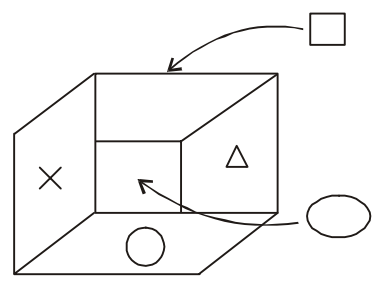##### Correct Option: D

According to question ,we can say that
The circle as at the bottom.1. Choose from the four answer figures, the figure that will be formed when the question figure is folded into a box.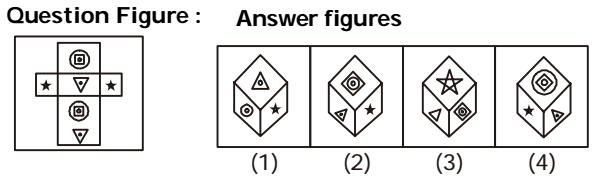1. On the basis of following pattern in given dices , we can say that
The question figure is similar to the Form ( 2 ).

##### Correct Option: B

On the basis of following pattern in given dices , we can say that
When the question figure is folded into a box it will look like answer figure (2).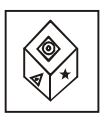1. In the figure you can see the picture of a group of blocks arranged in a particular way. All the blocks are of same size. You can see only some of the blocks in the group. Others are hidden by the blocks in front of them. Try to count the blocks in the group including those which you cannot see.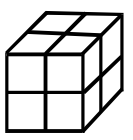1. On the basis of following pattern in given dices , we can say that
Seven block are visible and one block is invisible.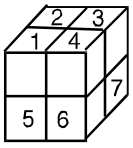##### Correct Option: B

On the basis of following pattern in given dices , we can say that
Seven block are visible and one block is invisible.
Total number of blocks = 7 + 1 = 8
Thus, there are 8 cubes.1. A cube is painted red on all the six sides. It is then cut into 27 equal cubes. How many cubes are coloured on one face only?

1. We follow the given pattern :-
On dividing in 27 parts there will be 3 parts of each edge.
One cube of each face will be coloured on only one face.

##### Correct Option: B

We follow the given pattern :-
On dividing in 27 parts there will be 3 parts of each edge.
One cube of each face will be coloured on only one face.
Therefore, 6 × 1 = 6

1. The figure above is a drawing of pile of Blocks. When taken apart, how many blocks would be there?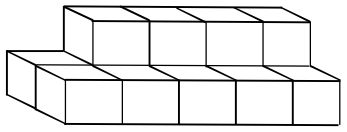1. On the basis of following pattern in given dices , we can say that
Ten block are visible and four block are invisible.

##### Correct Option: B

On the basis of following pattern in given dices , we can say that
Ten block are visible and four block are invisible.
From the diagram it is clear that there are 14 blocks.